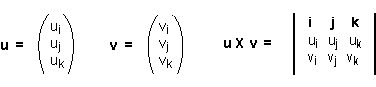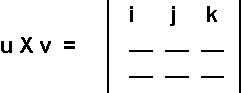What is: (1, 2, 3)T × (0, 0, 0)T ?

Obviously (1, 2, 3)T × 0 = 0

Or, plug into the formula u × v   =   ( uj vk - uk vj ,   uk vi - ui vk ,   ui vj - uj vi )T.

(1, 2, 3)T × (0, 0, 0)T   =   ( 2×0 - 3×0,   3×0 - 1×0,   1×0 - 2×0 )T  =   0

# Memory Aid

Here is a way to compute the cross product by arranging the elements of each vector into a determinant. This works as a memory aide, assuming you already know how to evaluate 3x3 determinants. If you don't know how, then skip this method and use the definition on the previous page.

The top row contains the symbols that stand for each axes. This is not the definition of cross product. In fact, it is not even a determinant. It is merely a memory aid.This only works for three dimensional vectors. (The cross product is not defined for vectors of any but three dimensions.) Put the first vector's components into the second row, and the second vector's components into the third row.

### QUESTION 12:

What is the cross product of (1, 2, 1)T with (0, -1, 2)T ?   Fill in the blanks: